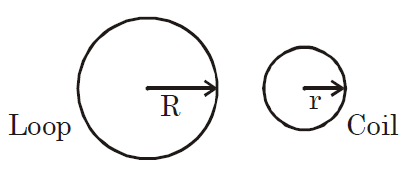# Q. One of the two identical conducting wires of length L is bent in the form of a circular loop and the other one into a circular coil of N identical turns. If the same current is passed in both, the ratio of the magnetic field at the central of the loop $(B_L)$ to that at the centre of the coil $(B_C)$ , i.e., $R \frac{B_L}{B_C}$ will be

JEE Main JEE Main 2019

Solution:

## $L = 2 \pi R L = N \times2\pi r$ $R =Nr B_{L} = \frac{\mu_{0} i}{2R}$ $B_{C} = \frac{\mu_{0} Ni}{2r} B_{C} = \frac{\mu_{0} N^{2} i}{2R}$ $\frac{B_{L}}{B_{C}} = \frac{1}{N^{2}}$You must select option to get answer and solution

## 1. The potential to which a conductor is raised, depends on ........

KCET 2005 Electrostatic Potential And Capacitance

## 2. The refractive index of a particular material is 1.67 for blue light, 1.65 for yellow light and 1.63 for red light. The dispersive power of the material is .........

KCET 2004 Electrostatic Potential And Capacitance

## 3. In a permanent magnet at room temperature

KCET 2019 Moving Charges And Magnetism

## 4. An antenna uses electromagnetic waves of frequency 5 MHz. For proper working, the size of the antenna should be

KCET 2019 Communication Systems

## 5. In the following circuit, what are P and Q?

KCET 2019 Semiconductor Electronics: Materials Devices And Simple Circuits

## 6. Two metal plates are separated by 2 cm. The potentials of the plates are − 10 V and + 30 V. The electric field between the two plates is

KCET 2019 Electrostatic Potential And Capacitance

KCET 2019 Nuclei

## 8. For a transistor amplifier, the voltage gain

KCET 2019 Semiconductor Electronics: Materials Devices And Simple Circuits

## 9. “Parsec” is the unit of

BHU 2007 Physical World, Units and Measurements

## 10. “Parsec” is the unit of

BHU 2007 Physical World, Units and Measurements